## Monday, November 30, 2009

### Quaternions -- Part 1: How many?

[Click here for a PDF of this post with nicer formatting or see below]
The Setup
Quaternions seem to be one of the least understood mathematical things amongst physicists. I have sat in countless lectures where at some point the lecturer pointed out that a particular topic could be understood or explained using quaternions, but, when pressed, could not really explain what, precisely, one of these quaternion thingies actually is.

The first encounter people have with quaternions is generally after they learn about the complex plane and its relationship to the regular 2D Cartesian plane. After seeing all sorts of nifty properties and uses of this relationship (we'll see one shortly) it's only natural to ask if there's a 3D complex analogue to the 2D complex plane. And, therefore, most books ask precisely this question. However, they usually give less-than-satisfactory attempts at generalizing, highlighting the mysterious algebraic problem of ``closure'' or something to that effect.

Then, often retelling the story of Hamilton and a bridge1 they pull some strange, ``4D'' quaternions out of a hat and show how they happily resolve all the algebraic problems. This, it seems, should be enough to placate even the most thoughtful reader, and stands in place of an actual explanation. And even though there is a lot of information about these buggers out there on the intertubes, all that I've seen is of the same approach.

So, it doesn't surprise me, honestly, that ``quaternion'' is also one of the most popular searches on this blog. The topic of quaternions is really too big to handle fully in one post (and, for full disclosure, I do not completely understand them myself), so this post will deal primarily with a rationale for the initial guess of a ``4D'' quaternion.

This post assumes you have read, and thoroughly grokked my discussion of dot and cross products, and have a solid understanding of traditional complex numbers.

Complex Numbers and 2D Vectors
My approach in this section is based on the fantastic book, Visual Complex Analysis by Tristan Needham. If something here isn't clear (and it's not the fault of my writing), or is different from the way you learned complex numbers, read this book. Even if everything is perfectly clear, read this book. What I'm trying to say is: Read this book.2

Recall that a complex number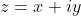can be represented by a vector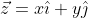in a 2D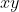-plane. Also, if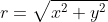-- i.e. the ``modulus'' of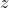or the length of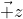-- and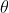is the ``argument'' ofor the angle betweenand the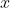-axis, we can also write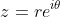in ``polar form.'' See  for pictures. We also have Euler's identity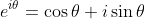(1)

Furthermore, recall that multiplying two complex numbers together effects a rotation and scaling. For example, multiplying a complex number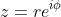-- graphically, a vector of length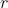making angle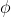with respect to the real axis (-axis) -- by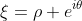gives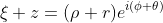. This can be understood graphically as a scaling ofby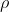and a rotation of the direction ofby the angle. Finally, the complex conjugate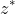ofis given either by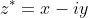or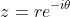.

From Complex Multiplication to Vector Products
For two complex numbers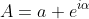and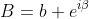let's see what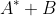is. This demonstration (at least initially) is based on .3 Anyway,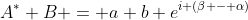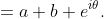(2)

Graphically this is a vector with length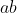at an angle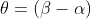from the-axis. Expanding this into a real and complex part using Euler's identity (1) gives: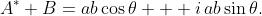(3)
We now note that the real part of this expression corresponds to the dot product between the two vectors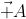and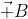. But should we do with the imaginary part?

Well, the magnitude of the imaginary part certainly corresponds to the magnitude of one dimension of the cross product between the two vectors. That is, if we relate the complex plane to the Cartesian-plane then the imaginary part ofis the-component of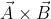. This important point is often lost in passing, and thus this property of complex multiplication is relegated to the realm of ``cool trick.'' However, we'll make good use of this detail.

Rethinking complex numbers
Now we are ready for the conceptual jump. Although we got to the representation of dot and cross products through use of a 2D complex plane, we're going to distance ourselves from this wonderful visualization for the moment and note that an arbitrary complex number has two parts: One corresponds to a dot product, the other corresponds to one dimension of a cross product of two vectors.4 If we want to find a relationship between complex numbers and 3D vectors we need to pick one of these parts to generalize.

Now, recall that the dot product yields a scalar quantity equal to the amount that two vectors point in the same direction. Since there is no directionality or dimensionality inherent in this quantity -- it's just a length -- there's really no way to add extra bits here. Length stays a scalar in any dimension.

So, instead we turn to the cross-product part. In the preceding section I repeatedly stressed that the imaginary part of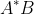corresponds to one dimension of a 3D cross product. However, which single dimension of the cross product we choose is completely arbitrary: Just as with the calculation of area for the cross product, the 2D Cartesian plane we choose to map to the complex plane could just as easily be the-,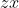- or the-planes.

Recall, that to resolve this ambiguity in in cross-product land we chose to identify which plane we were talking about by a right-hand rule normal vector to the plane. However, here we're attempting to generalize complex numbers, not cross products per se. So, instead of assigning different normal vectors to each cross product term, let's assign a different complex number to each term. That is,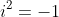and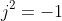, but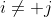for example. Then, we assignto the cross product of two vectors in the-plane andto the cross product of two vectors in the-plane.

The one question remaining, though, as we generalize our complex plane, is how many additional complex numbers do we need? Maybe, naively, we can try adding just one extra cross-product dimension. That is,andonly. The problem, though, can be seen in cross-product land.

Closure
Remember, that a cross product resultant vector is a normal vector to an arbitrary plane in 3D Cartesian space, and thus always requires all three unit vectors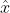,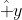and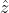. For example, the cross product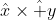is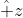. That is, in order to make sense of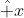crosswhich can exist in 2D, you must already have a third unit vector.

Physically, in Cartesian vector space, it means that you must be able to add any arbitrary 3D cross product resultant vectors and still get a 3D vector. In fact, if this wasn't true, there'd be no way to even write the 3D cross product in the first place since you need to project the arbitrary vectors to three (independent) 2D planes and then add the resulting normal vectors. You can't have just two cross-product parts and get a result that always makes sense. This is the requirement of ``closure.''

The reason there's no problem in the 2D plane version is simply because there's only one possible normal vector, so we only look at the magnitude of the cross product -- i.e. the amount of area -- and the sign. And that is just a scalar! In 2D land nothing is preventing you from adding the cross product to the dot product -- they're both scalars -- so you can write a two-element complex numbercombination with no trouble.

However, in 3D we can't simply add a vector to a scalar, and therefore we need all three parts of the cross product. So too, then, if we want a generalized complex number to have a dot-product part and a cross-product part that makes physical sense, we need three complex numbers:andfrom above, plus a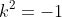,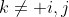corresponding to the cross product of the projection of vectors in the-plane.

Thus, we now have a generalized complex number -- quaternion -- of the form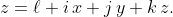(4)

References
 E. Lansey. The dot product and cross products [online]. April 2009. Available from: http://behindtheguesses.blogspot.com/2009/04/dot-and-cross-products.html.
 Tristan Needham. Visual Complex Analysis. Oxford University Press, Oxford, UK, 1997.
 C. Doran and A.N. Lasenby. Geometric algebra for physicists. Cambridge University Press New York, Cambridge, UK, 1st edition, 2003.

1 Just Google it, it's not really worth retelling, in my opinion.
2 If I was stranded on an island forever but could bring only one math book, this would be it.
3 Just go out and get that book already! What are you waiting for?
4Thanks, Peeter, for recommending the book () which highlighted this point.